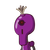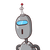# the angle of a quadrilaterial are in the ratio 1:2:3:4 state the measure of its angle.​

the angle of a quadrilaterial are in the ratio 1:2:3:4 state the measure of its angle.​

### 2 thoughts on “the angle of a quadrilaterial are in the ratio 1:2:3:4 state the measure of its angle.​”

1.The figure can be as follows: Now, it is very well known that the sum of all the interior angles of the quadrilateral is 360 degrees. So here the angles will be x=36∘, 2x=72∘, 3x=108∘, and 4x=

2.Step-by-step explanation:

the Sume of all the angles in quadrilateral is 360°

so

x+2x+3x+4x=360

10x=360

x=36°

so

2x=72

3x=108

4x=144

these are the measures of the angles

hoping it helps

mark as brainliest